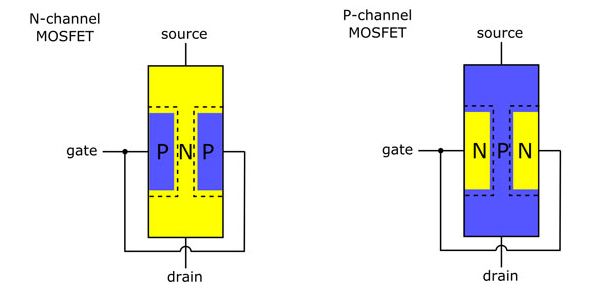# Gibilisco: Field Effect Transistor! Quiz

20 Questions | Total Attempts: 1681SettingsDo you know anything about Gibilisco: Field Effect Transistor? It is a type of transistor that employs an electric field to manage the flow of current. It is contained by the changes in the surface potential caused by the binding of molecules. This quiz will need to understand things like what a JFET is and what transconductance is. This quiz will show you just how much you realize about the field effect transistor.

• 1.
The current through the channel of a JFET is directly affected by all of the following, except the
• A.

Drain voltage.

• B.

Transconductance.

• C.

Gate voltage.

• D.

Gate bias.

• 2.
In an N-channel JFET, pinchoff occurs when the gate bias is
• A.

Small and positive.

• B.

Zero.

• C.

Small and negative.

• D.

Large and negative.

• 3.
The current consists mainly of holes when a JFET
• A.

Has a P-type channel.

• B.

Is forward-biased.

• C.

Is zero-biased.

• D.

Is reverse-biased.

• 4.
A JFET might work better than a bipolar transistor in
• A.

A high-voltage rectifier.

• B.

A weak-signal RF amplifier.

• C.

A power-supply filter.

• D.

A power transformer.

• 5.
In a P-channel JFET,
• A.

The drain is forward-biased.

• B.

The source-gate junction is forward-biased.

• C.

The drain is negative relative to the source.

• D.

The gate must be at dc ground.

• 6.
A JFET is sometimes biased at or beyond pinchoff in
• A.

A power amplifier.

• B.

A rectifier.

• C.

A filter.

• D.

A weak-signal amplifier.

• 7.
The gate of a JFET exhibits a
• A.

Forward bias.

• B.

High impedance.

• C.

Low reverse resistance.

• D.

Low avalanche voltage.

• 8.
Which of the following conditions is not normally desirable in a JFET?
• A.

A conducting channel

• B.

Holes as the majority carriers

• C.

A forward-biased P-N junction

• D.

A high input impedance

• 9.
When a JFET is pinched off,
• A.

The value of dID/dEG is very large with no signal input.

• B.

The value of dID/dEG might vary considerably with no signal input.

• C.

The value of dID/dEG is negative with no signal input.

• D.

The value of dID/dEG is zero with no signal input.

• 10.
Transconductance is the ratio of
• A.

A change in drain voltage to a change in source voltage.

• B.

A change in drain current to a change in gate voltage.

• C.

A change in gate current to a change in source voltage.

• D.

A change in drain current to a change in drain voltage.

• 11.
Characteristic curves for JFETs generally show
• A.

Drain voltage as a function of source current.

• B.

Drain current as a function of gate current.

• C.

Drain current as a function of drain voltage.

• D.

Drain voltage as a function of gate current.

• 12.
A disadvantage of MOS components is the fact that
• A.

They can be easily damaged by static electricity.

• B.

They need a high input voltage in order to amplify.

• C.

They draw large amounts of current.

• D.

They produce a great deal of heat.

• 13.
The input impedance of a MOSFET is
• A.

Lower than that of a JFET.

• B.

Lower than that of a bipolar transistor.

• C.

Between that of a bipolar transistor and a JFET.

• D.

Extremely high.

• 14.
A significant difference between MOSFETs and JFETs is the fact that
• A.

MOSFETs can handle a wider range of gate bias voltages.

• B.

MOSFETs can deliver greater output power.

• C.

MOSFETs are more rugged.

• D.

MOSFETs last longer.

• 15.
The channel in a zero-biased JFET is normally
• A.

Pinched off.

• B.

In a state of avalanche breakdown.

• C.

In a conducting state.

• D.

• 16.
When an enhancement-mode MOSFET is at zero bias,
• A.

The drain current is high with no signal.

• B.

The drain current fluctuates with no signal.

• C.

The drain current is low with no signal.

• D.

The drain current is zero with no signal.

• 17.
An enhancement-mode MOSFET can be recognized in schematic diagrams by the presence of:
• A.

An arrow pointing inward.

• B.

A broken vertical line inside the circle.

• C.

An arrow pointing outward.

• D.

A solid vertical line inside the circle.

• 18.
In a source follower, which of the electrodes receives the input signal?
• A.

Any of them (doesn’t matter)

• B.

The source

• C.

The gate

• D.

The drain

• 19.
Which of the following circuits produces an output signal wave that is 180° out of phase with the input signal wave?
• A.

The common source circuit

• B.

The common gate circuit

• C.

The common drain circuit

• D.

All of the above

• 20.
Which of the following circuits can produce the greatest signal gain (amplification factor)?
• A.

The common source circuit

• B.

The common gate circuit

• C.

The common drain circuit

• D.

All of the above circuits can amplify to the same extent.

Related TopicsBack to top